# IF AND in ExcelArticle byExcelMojo Team## What Is IF AND Function In Excel?

The IF AND in Excel includes two logical functions, AND and IF, that checks for multiple criteria simultaneously. The AND() output decides whether the IF() output should be the TRUE or FALSE value.

Users can use the IF AND function when purchasing a stock or updating a status based on more than one condition.

For example, the below table contains six teams’ scores in two rounds of a quiz competition. We must decide the teams qualifying for the third round based on their previous two rounds’ scores using the IF AND function. Assume the qualifying score for the two rounds is 45.

Select cell D2, enter the formula =IF(AND(B2>=45,C2>=45),”Qualified”,”Not Qualified”), press “Enter”, and drag the formula from cell D2 to D7 using the fill handle.

[Output Observation: In the above example, the AND excel function checks if the scores in the two rounds equal or exceed 45 for each team. And if both criteria hold, the AND() output is TRUE. If the IF condition is TRUE, it returns “Qualified” as the status for the corresponding team. Otherwise, the output is “Not Qualified”. Column E is for our reference.]

###### Key Takeaways
• The IF AND in Excel is a formula that uses the AND() to check multiple criteria in one go. And based on whether the AND function returns TRUE or FALSE, the IF function output will be the TRUE or FALSE value.
• Users can apply the IF AND formula in Excel to check multiple conditions. For instance, the function helps decide whether to buy a commodity based on its price and discount percentage.
• We can use the formula as it is or in different forms, such as “nested IF AND”and “IF-ANDOR”, and with more than two conditions.

### IF AND() Excel Formula

The syntax for IF ANDExcel Formula is,

And we can enter it in an Excel cell as:

The arguments for IF AND Excel Formula are,

• logical_test: It is the AND(), which returns TRUE if all the specified conditions hold.
• value_if_true: The TRUE value the IF() should return if the logical_test condition is TRUE.
• value_if_false: The FALSE value the IF() should return if the logical_test condition is FALSE.

[Note: The above formula of the IF() shows the last two arguments as optional. The logical_test (AND()) and the value_if_true arguments are mandatory, and only the third argument is optional in the If And Excel statement.]

### How To Use IF AND Excel Function?

We can use If And in Excel in 2 ways, namely,

1. Access from the Excel ribbon.
2. Enter in the worksheet manually.

#### Method #1 – Access from the Excel ribbon

Choose a target cell for output → select the “Formulas” tab → go to the “Function Library” group → click the “Logical” option drop-down → select the “IF” or “AND” function, as shown below.

The Function Arguments window appears. Enter the arguments in the “Logical_test, Value_if_true, and Value_if_false” fields → click OK, as shown below.

#### Method #2 – Enter in the worksheet manually

1. Choose a target cell for the output.
2. Type =IF(AND() in the cell. [Alternatively, type =I, double-click the IF function from the Excel suggestions, type A, and double-click the AND function from the Excel suggestions.]
3. Enter the arguments as cell values or references and close the brackets.
4. Press Enter to execute and get the required outcome.

The below table shows the order delivery details for a list of items. We must check if the total units delivered meet the minimum required number of units, and if the order delivery is before April for each item. And if the two conditions hold, the order status is complete. Otherwise, the status is pending.

The steps to use IF AND in Excel function in order to check delivery status are,

1. Select cell E2, and enter the formula

=IF(AND(C2>=B2,MONTH(D2)<4),”Complete”,”Pending”)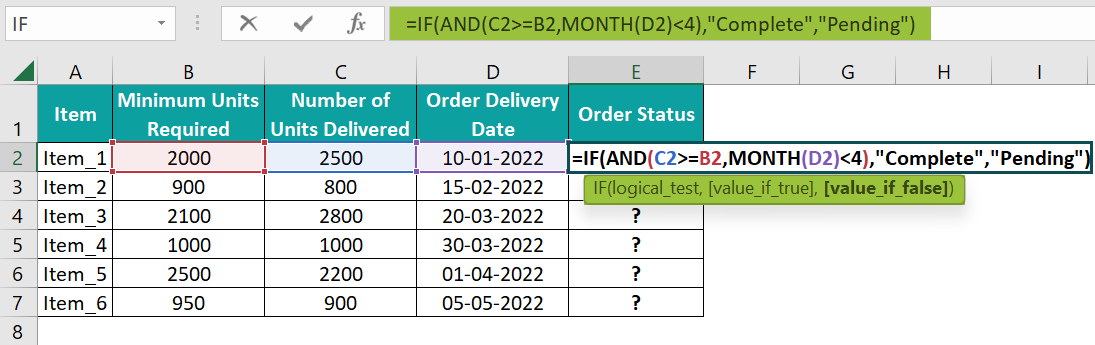2. Press Enter to view the order status.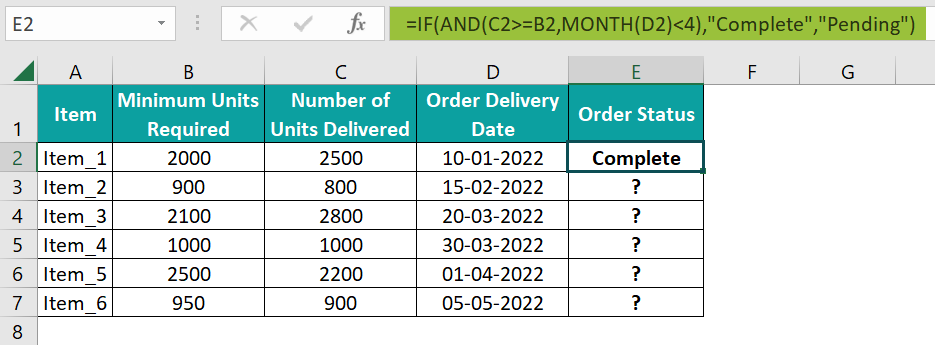[Alternatively, select the target cell E2 and go to FormulasLogicalIF to apply the function in the chosen cell.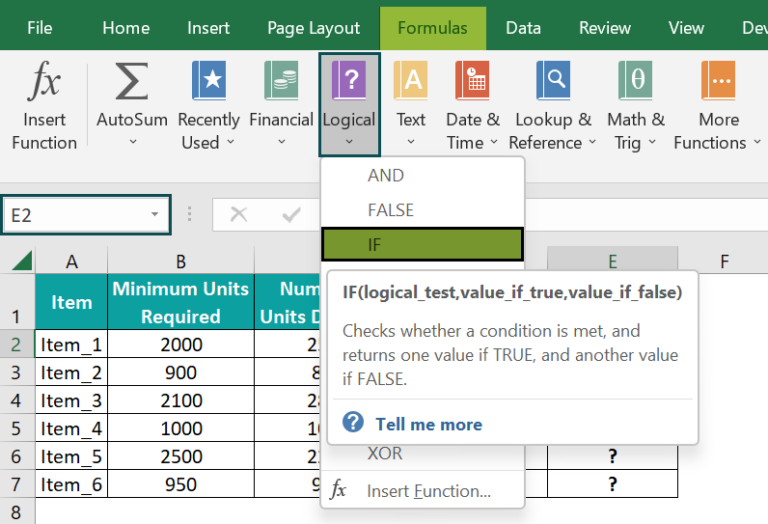The above step will open the Function Arguments window. Enter the required conditions within the AND() and the IF() TRUE and FALSE values in the respective fields.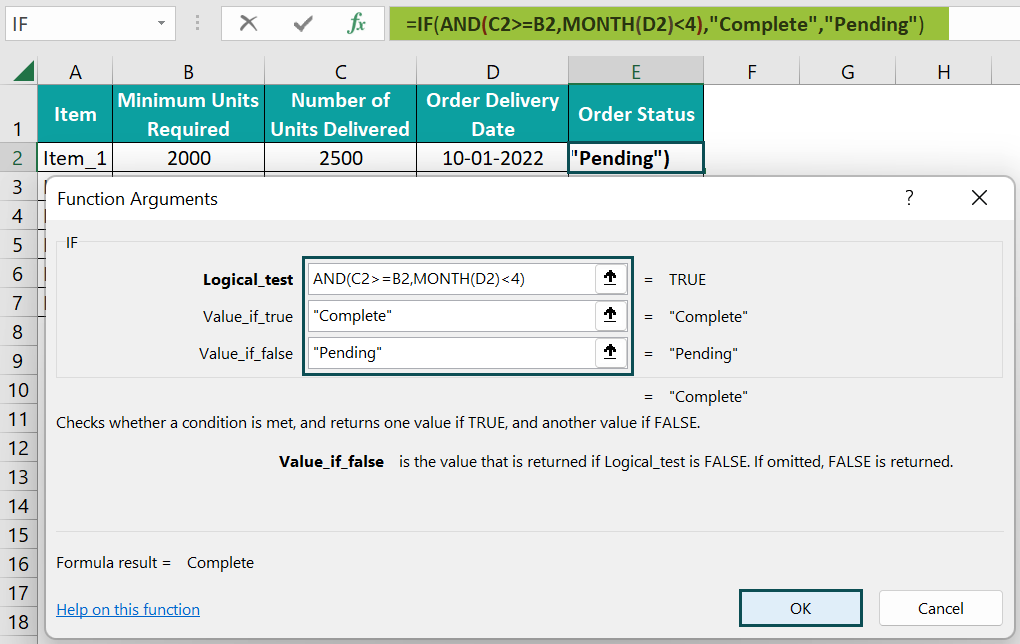Finally, clicking OK will close the window, and we can see the IF AND formula output.]

3. Using the fill handle, drag the formula in cells E3:E7.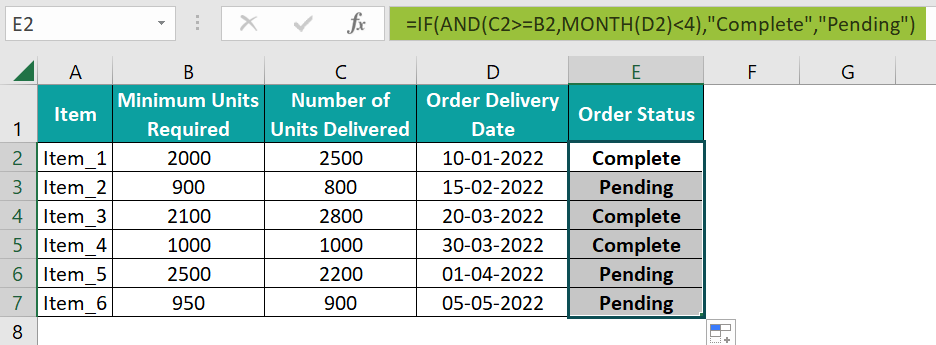[Output Observation: Let us consider the cell E7 expression to see how the formula works.

First, the AND() checks the two given conditions.

The first one is whether the number of units delivered, 900, exceeds the required minimum units of 950. And the second condition checks if the MONTH() output, the month of the specified date, 5 (a value indicating May), is less than 4 (a value indicating April).

But, as both criteria are FALSE, the AND() output is FALSE. Thus, the IF condition does not hold, and the IF() returns the FALSE value, Pending, as the Item_6 order status.]

### Examples

We will consider some advanced scenarios using the IF AND in Excel examples.

#### Example #1

The following table shows a list of branded items and their costs. Suppose, the store decides to give a 20% discount on items of the Gap brand that are less than \$30. Otherwise, the discount percentage is 5%.

The steps to calculate using the IF AND in Excel are,

• Step 1: Select the cell range C2:C7, go to HomeNumber Format, and set the data type as Currency.
• Step 2: Select cell C2, enter the formula=IF(AND(A2=”Gap”,B2<30),B2*20%,B2*5%), and press Enter. The result is \$2.50, as shown below.
• Step 3: Using the excel fill handle, drag the formula in the remaining target cells C3:C7.

[Output Observation: Let us check the cell C7 expression to learn how the formula executes.

The AND() checks if the brand specified in cell A7 is Gap and the item cost is less than 30. And as both conditions are TRUE, the AND() output is TRUE. Therefore, the IF condition holds, and the IF function calculates and returns 20% of the cell B7 value, \$5.]

#### Example #2

The following illustration explains how to apply the IF and AND function in Excel with multiple conditions.

The below table shows a list of students, their overall aggregates, attendance data, and project submission status. We must update their scholarship eligibility, and the eligibility criteria are that the aggregate should be 450 marks or above, and attendance must be 90 days or above. The project submission status should be Yes.

The steps to apply the IF and AND function in Excel with multiple conditions are,

• Step 1: Select cell E2, enter the formula =IF(AND(B2>=450,C2>=90,D2=”Yes”),”Eligible”,”Not Eligible”), and press Enter. The result is “Eligible”, as shown below.
• Step 2: Drag the formula from cell E2 to E11 using the fill handle.

[Output Observation: Let us take the cell E11 expression to check how the formula works.

The AND() checks if Jane Francis’s aggregate, 458, is greater or equal to 450, her attendance, 101 days, is greater or equal to 90 days, and she has submitted the project.

And as all the criteria hold, the AND() output is TRUE, implying that the IF condition is TRUE. So, the IF() returns the TRUE value, Eligible, as Jane Francis’s scholarship edibility.]

#### Example #3

We shall see an example of nested IF AND in Excel.

The below table contains a list of employees, their designations, and utilization data.

The requirement is to update the performance rating for each employee in column D based on their designation and utilization. The conditions are:

• Performance Rating will be “Exceeds Expectations” for Designation: Senior Engineer, Utilization: >=95%.
• Performance Rating will be “Meets Expectations” for Designation: Senior Engineer, Utilization: >=80% and <95%.
• Performance Rating will be “Under Performer” for Designation: Senior Engineer, Utilization: <80%.

The steps to apply the nested IF AND in Excel are,

• Step 1: Select the target cell D2, enter the below formula, and press Enter.

=IF(AND(B2=”Senior Engineer”,C2>=95%),”Exceeds Expectations”,IF(AND(B2=”Senior Engineer”,C2>=80%,C2<95%),”Meets Expectations”,IF(AND(B2=”Senior Engineer”,C2<80%),”Under Performer”,”NA”)))

• Step 2: Using the fill handle, drag the formula to cells D3:D11.

[Output Observation: Let us see the cell D11 expression to know how the formula executes.

First, the AND() in the outer IF() checks if Tracy Bridges is a Senior Engineer and whether her utilization isgreater or equal to 95%. But as the utilization percentage condition does not hold, the AND() output is FALSE, implying the outer IF condition does not hold.

So, the first inner IF(), the outer IF() FALSE value, executes. The AND() checks if the employee is a Senior Engineer and their utilization is between 80% to 95%. In this case, the AND() returns TRUE as both conditions hold. So, the IF() returns the TRUE value, Meets Expectations, as the performance rating of Tracy Bridges.]

### Important Things To Note

• In the IF AND in Excel, supplying the AND() as the logical_test argument and the value_if_true argument to the IF excel function is mandatory.
• The Excel IF AND formula is case-insensitive.
• We can evaluate 255 conditions using the AND() in the IF AND formula.
• Suppose the AND() in the IF AND function cannot return a logical value. Then the formula output will be the #VALUE! error.

1. Is there an IF AND function in Excel?

There is no inbuilt If And function in Excel. However, we can apply the below IF AND formula, which works using two logical functions, IF and AND.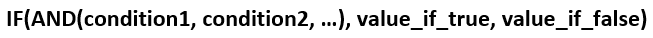The condition arguments inside the AND() (the IF condition) are the multiple criteria that the function must check. The two arguments in the IF() are the TRUE and FALSE values that the function returns based on the AND() output.

2. When to use the IF ANDfunction in Excel?

For example, the function is useful when deciding whether a venture is pursuable based on multiple criteria such as its yearly turnover, profit margin, and scope of expansion.

3. Can you use the IF AND formula with the OR function in Excel?

We can use the IF AND formula with the OR function in Excel.

Let us see the steps with an example.

The table below lists laptop models, their prices, and their colors. We must update the availability status for each laptop in column D, but the conditions are that the status should show as “Available” only if the laptop price is equal to or greater than \$500. And the laptop’s color is Black, Platinum Blue, or White.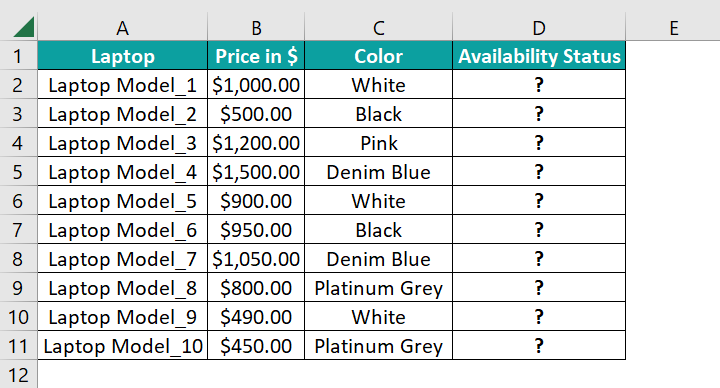The steps to apply the IF AND formula with the OR function are,

• Step 1: Select the target cell D2, enter the below formula, and press Enter.

=IF(AND(B2>=500,OR(C2=”Black”,C2=”Platinum Grey”,C2=”White”)),”Available”,”Not Available”)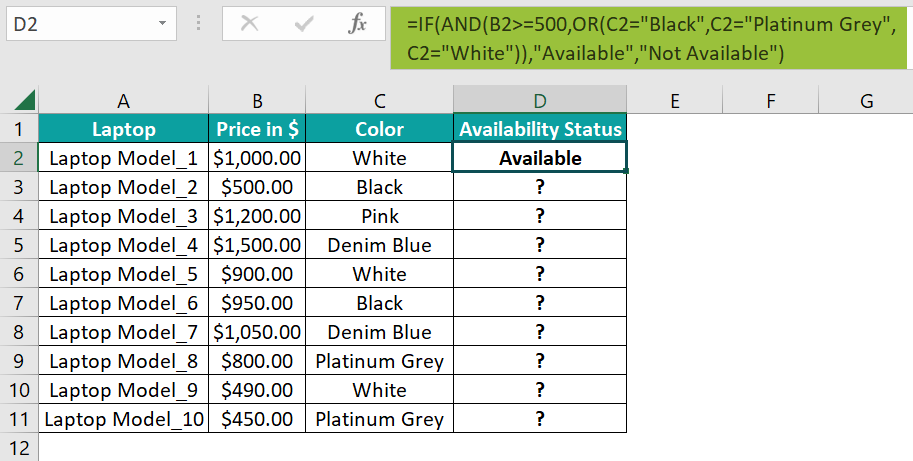• Step 2: Drag the formula from cell D2 to D11 using the fill handle.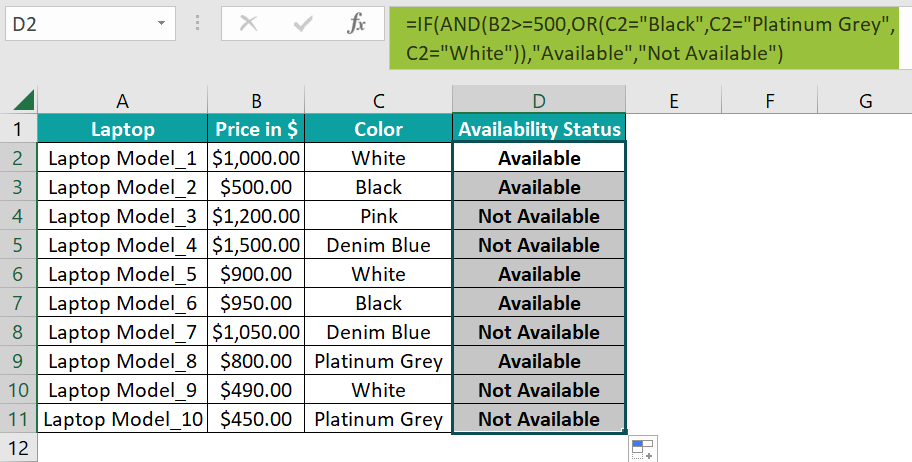[Output Observation: Let us see the cell D11 expression to understand how the formula works.

First, the OR() checks if the cell C11 value is any of the specified colors. And as the second criterion in the OR() holds, the OR() returns TRUE. Next, the AND() checks if the laptop price equals or exceeds \$500. But as the given rate is \$450, the condition does not hold.

So, as not all the conditions in the AND() hold, the AND() output is FALSE. Thus, the IF condition does not hold, leading to the IF() returning the FALSE value, “Not Available”, as the Laptop Model_10 availability status.]